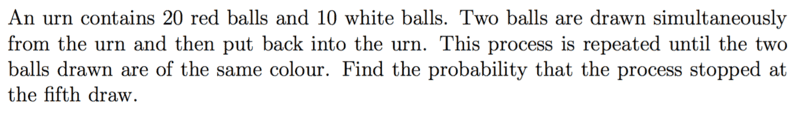# Probability Theory: Simultaneous picks

## Homework Statement

[/B]
Hi all, I have an issue understanding the concepts pertaining to the following problem, assistance is greatly appreciated.I understand the "flow" of the problem; first find the probability of obtaining balls of the same colour, then use the geometric distribution. However, I don't understand why the method of counting probabilities doesn't change between the cases of successive picks, and simultaneous picks.

The way I always understood outcome-counting for successive picks (number of ways of picking 2 red balls w/o replacement for instance) was that the first pick had 20 available options, the second 19. Then divide by 2 to account for the fact that we don't bother with order, so
$$\frac{20\times 19}{2} = {20\choose2}$$
The case of simultaneous picks doesn't come as intuitively to me, I have tried to find ways to understand it (like "how many ways can i touch 20 red balls with my 2 hands") but every time it makes sense, it doesn't.

Could anyone assist? Many thanks!